Next: Expectation Values and Variances Up: Fundamentals of Quantum Mechanics Previous: Schrödinger's Equation

# Normalization of the Wavefunction

Now, a probability is a real number between 0 and 1. An outcome of a measurement which has a probability 0 is an impossible outcome, whereas an outcome which has a probability 1 is a certain outcome. According to Eq. (138), the probability of a measurement ofyielding a result between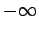andis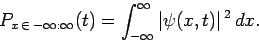(139)

However, a measurement ofmust yield a value betweenand, since the particle has to be located somewhere. It follows that, or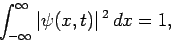(140)

which is generally known as the normalization condition for the wavefunction.

For example, suppose that we wish to normalize the wavefunction of a Gaussian wave packet, centered on, and of characteristic width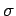(see Sect. 3.12): i.e.,(141)

In order to determine the normalization constant, we simply substitute Eq. (141) into Eq. (140), to obtain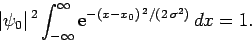(142)

Changing the variable of integration to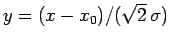, we get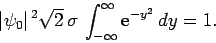(143)

However,(144)

which implies that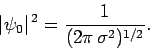(145)

Hence, a general normalized Gaussian wavefunction takes the form(146)

whereis an arbitrary real phase-angle.

Now, it is important to demonstrate that if a wavefunction is initially normalized then it stays normalized as it evolves in time according to Schrödinger's equation. If this is not the case then the probability interpretation of the wavefunction is untenable, since it does not make sense for the probability that a measurement ofyields any possible outcome (which is, manifestly, unity) to change in time. Hence, we require that(147)

for wavefunctions satisfying Schrödinger's equation. The above equation gives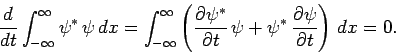(148)

Now, multiplying Schrödinger's equation by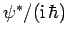, we obtain(149)

The complex conjugate of this expression yields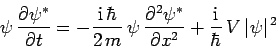(150)

[since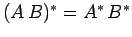,, and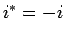]. Summing the previous two equations, we get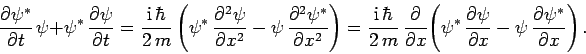(151)

Equations (148) and (151) can be combined to produce(152)

The above equation is satisfied provided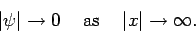(153)

However, this is a necessary condition for the integral on the left-hand side of Eq. (140) to converge. Hence, we conclude that all wavefunctions which are square-integrable [i.e., are such that the integral in Eq. (140) converges] have the property that if the normalization condition (140) is satisfied at one instant in time then it is satisfied at all subsequent times.

It is also possible to demonstrate, via very similar analysis to the above, that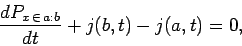(154)

where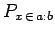is defined in Eq. (138), and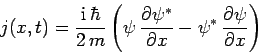(155)

is known as the probability current. Note thatis real. Equation (154) is a probability conservation equation. According to this equation, the probability of a measurement oflying in the intervaltoevolves in time due to the difference between the flux of probability into the interval [i.e.,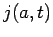], and that out of the interval [i.e.,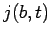]. Here, we are interpreting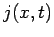as the flux of probability in the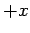-direction at positionand time.

Note, finally, that not all wavefunctions can be normalized according to the scheme set out in Eq. (140). For instance, a plane wave wavefunction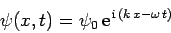(156)

is not square-integrable, and, thus, cannot be normalized. For such wavefunctions, the best we can say is that(157)

In the following, all wavefunctions are assumed to be square-integrable and normalized, unless otherwise stated.Next: Expectation Values and Variances Up: Fundamentals of Quantum Mechanics Previous: Schrödinger's Equation
Richard Fitzpatrick 2010-07-20